# subtracting Fractions Problems

#### Number of problems found: 91

• Add sub fractionsWhat is 4 1/2+2/7-213/14?
• Length subtractingExpress in mm: 5 3/10 cm - 2/5 mm
• Pizza fractionsAnn ate a third of a pizza and then another quater. Total part of pizza eaten by Ann and how much pizza is left?
• SchoolThere are 150 pupils in grade 5 . 2/3 of them are female. By what fractions are the males?
• Fractions mul add sumTo three-eighths of one third, we add five quarters of one half and multiply the sum by four. How much will we get?
• Difference mixed fractionsWhat is the difference between 4 2/3 and 3 1/6?
• Cake fractions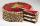Thomas ate 1/3 of cake, Bohouš of the rest of the cake ate 2/5. What fraction of cake left over for others?
• Pounds3 pounds subtract 1/3 of a pound.
• EmployeesOf all 360 employees, there are 11/12 women. How many men work in a company?
• Package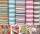The package was 23 meters of textile. The first day sold 12.3 meters. How many meters of textile remained in the package?
• FortyForty five of the 80 students were girls. What is the ratio of girls to boys?
• Find the 24Find the difference between 2/7 and 1/21
• Coloured teacups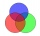The teacups in Tea Stop 55 are `2/5` green and `3/10` yellow. What fraction of the teacups are neither green nor yellow?
• Akpan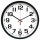Akpan spent 3/8 of his time in school during the week. What fraction of his time does he spend at home during the week?
• Pupils 7There are 40 pupils in a certain class. 3/5 of the class are boys. How many are girls?
• MichaelMichael had a bar if chocolate. He ate 1/2 of it and gave away 1/3. What fraction had he left?
• A jewelry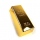A jewelry store has 20 grams of gold. If a pair of earrings need 1/4 gram of gold, how many grams are not used?
• RegroupingSubtract mixed number with regrouping: 11 17/20- 6 19/20
• A 14.5-gallonA 14.5-gallon gasoline tank is 3/4 full. How many gallons will it take to fill the tank? Write your answer as a mixed number.
• Two pizzasJacobs mom bought two whole pizzas. He ate 2/10 of the pizza and his dad ate 1 1/5. How much is left.

Do you have an interesting mathematical word problem that you can't solve it? Submit a math problem, and we can try to solve it.

We will send a solution to your e-mail address. Solved examples are also published here. Please enter the e-mail correctly and check whether you don't have a full mailbox.

Please do not submit problems from current active competitions such as Mathematical Olympiad, correspondence seminars etc...
Need help to calculate sum, simplify or multiply fractions? Try our fraction calculator. Subtracting Problems. Fraction Word Problems.